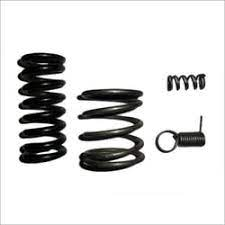• +(91) 9290170008
Coil spiral springs manufacturer in India

## Spiral Spring/Flat Spring/Torsion Spring manufacturers in India

Bhalla Fasteners is the leading manufacturer, supplier and exporter of coil spring to USA,UK,Poland,Germany,France,Italy,Finland,Netherlands and other european countries Bhalla Fasteners also manufactures top quality compression spring which acts as a shock absorber in suspension parts of cars and trucks.
A coiled spring can be used in automotive coil springs also.
Bhalla Fasteners have been able to offer Spiral Spring, Flat Spring, Torsion Springs, in various ranges and models.

Their Spiral Spring, Flat Spring, Torsion Springs, position in the international market, they are best in providing Spiral Spring, Flat Spring, Torsion Springs, to the regular clients at very inexpensive priceWeight Table

## Calculate Compression Spring Weight

#### Calculate Wire Length

• 1.- Determine Mean Diameter (D) Add one wire diameter to the inner diameter or subtract one wire diameter from the outer diameter.
• 2.- Multiply mean diameter (D) by 3.1415 (Pi). This gives you the length of one coil in inches.
• 3.- Multiply #2 by total number of coils (N)
• 4.-This figure equals the length in inches of this spring.
##### To Determine the Total Feet in the Job
• 5.- Multiply #4 by the number of parts in the job (the quantity)
• 6.- Divide #5 by 12 to get the total feet in the job and record this number

#### Wire Length Formula#### Calculate Spring Weight

• 7.- Wire diameter x wire diameter
• 8.- #7 multiplied by 9.4245
• 9.- #8 multiplied by the density (MW / HD = 0.290, SST = 0.291, BerCu / PhBrz = 0.302)
• 10.- #9 multiplied by #6 = Total Pounds in Job
##### To Determine the Number of Feet Per 1,000 Springs
• 11.- Multiply #4 by 1,000
• 12.- Divide this figure by 12

#### Spring Weight#### Calculate Wire Length

• 1.- Determine Mean Diameter (D) Add one wire diameter to the inner diameter or subtract one wire diameter from the outer diameter.
• 2.- Multiply mean diameter (D) by 3.1415 (Pi). This gives you the length of one coil in inches.
• 3.- Multiply #2 by total number of coils (N) add two coils (hooks).
• 4.- This figure equals the number of inches in the spring.
##### To Determine the Total Feet in the Job
• 5.- Multiply #4 by the number of parts in the job. (the quantity)
• 6.- Divide #5 by 12 to get the total feet in the job and record this number.

#### Wire Length Formula#### Calculate Spring Weight

• 7.- Wire diameter x wire diameter
• 8.- #7 multiplied by 9.4245
• 9.- #8 multiplied by the density (MW / HD = 0.290, SST = 0.291, BerCu / PhBrz = 0.302)
• 10.- #9 multiplied by #6 = Total Pounds in Job
##### To Determine the Number of Feet Per 1,000 Springs
• 11.- Multiply #4 by 1,000
• 12.- Divide this figure by 12

#### Spring Weight#### Calculate Wire Length

• 1.- Determine Mean Diameter (D) Add one wire diameter to the inner diameter or subtract one wire diameter from the outer diameter.
• 2.- Multiply mean diameter (D) by 3.1415 (Pi). This gives you the length of one coil in inches.
• 3.- Multiply #2 by total number of coils (N)
• 4.- Add both leg lengths after doing steps #1 through #3 to finalize the calculation of inches in one spring.
##### To Determine the Total Feet in the Job
• 5.- Multiply #4 by the number of parts in the job (the quantity)
• 6.- Divide #5 by 12 to get the total feet in the job and record this number

#### Wire Length Formula#### Calculate Spring Weight

• 7.- Wire diameter x wire diameter
• 8.- #7 multiplied by 9.4245
• 9.- #8 multiplied by the density (MW / HD = 0.290, SST = 0.291, BerCu / PhBrz = 0.302)
• 10.- #9 multiplied by #6 = Total Pounds in Job
##### To Determine the Number of Feet Per 1,000 Springs
• 11.- Multiply #4 by 1,000
• 12.- Divide this figure by 12

#### Spring WeightSpecification Table## Coil springs manufactures, exporters and suppliers in India

Bhalla Fasteners is the leading manufacturer, supplier and exporter of coil spring to USA,UK,Poland,Germany,France,Italy,Finland,Netherlands and other european countries. Great quality Conical Springs are designed and developed under the guidance of our skilled engineers utilizing premium grade raw materials. At Bhalla fasteners the entire range of Conical Springs is highly demanded in the global market for its optimum strength, high durability, corrosion resistance, robust construction, ease at installation and durability.